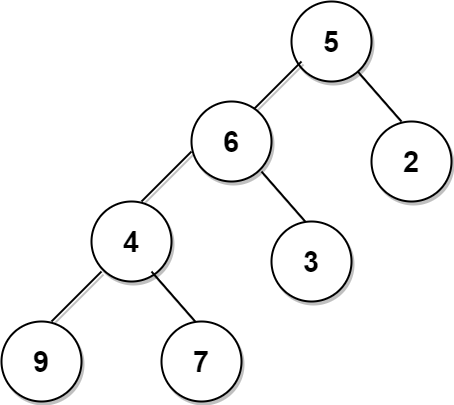# Find the Maximum Path Sum Between Two Leaves of A Binary Tree

## Introduction.

Binary trees are the specialized version of generic trees where every node can have at most only two children named as the left child and right child of a binary tree. In this blog, we will be discussing a problem where we have to find out the maximum possible sum of the path between any two leaves of a given binary tree. Let us understand this problem clearly with the below example:

Let us find the path sum between all leaves of this binary tree and then we will select the maximum among them as our output.Out of all the above six paths, path 9->4->6->5->2 is the maximum sum path with a sum of 26. Hence the output will be 26.

I hope the problem is crystal clear to you now. So now is the time to move towards its solution.

## Method 1:

In this approach, for every node we will be finding out the maximum sum node-to-leaf path from the left and right subtrees:

Step 1: We’ll be finding the maximum sum from the current node to a leaf node in the left subtree of the traversed node.

Step 2: Likewise, find the maximum sum from the current node to a leaf node in the right subtree of the traversed node.

Step 3: Add the values obtained in steps 1 and 2 and also add the data of the traversed node to get the maximum sum node-to-leaf path using the left and right subtrees.

Step 4: Compare it with the maximum value obtained so far and update the maximum value.

Let’s see this approach in code:

## Complexity Analysis:

Time Complexity: The time complexity is O(N^2) where N is the number of nodes in the binary tree. For every node in the tree, we are calculating the maximum sum node-to-leaf path of its left subtree and right subtree. This takes O(N) time and since there are ‘N’ nodes, the final complexity is O(N * N) = O(N^2).

Space Complexity: The space complexity will be O(N), where N is the number of nodes in the binary tree.

The recursive stack will take O(H) space where H is the height of the tree. In the worst case, H will be equal to N, when each node in the tree has only one child. Thus, the space complexity is O(N).

## Method 2:

In this approach, we will be getting the maximum path sum of a binary tree in a single traversal of the binary tree. Let’s see the steps to this problem:

Step 1: Start from the bottom of the tree and return each node's maximum sum node-to-leaf path to its parent node.

Step 2: Calculate the maximum sum path between the leaves of left and right subtrees rooted at each node and update the maximum sum during the traversal in the variable named maxPathSum.

At the end of these steps, you will be having your answer in the maxPathSum sum variable.

You will understand this approach better by going through the below code.

## Complexity Analysis:

Time Complexity: The time complexity of this approach will be O(N) , where N is the number of nodes in the binary tree.

Since we are traversing the entire tree only once, the final complexity is O(N).

Space Complexity: The space complexity will be O(N), where N is the number of nodes in the binary tree.

The recursive stack will take O(H) space where H is the height of the tree. In the worst case, H will be equal to N, when each node in the tree has only one child. Thus, the space complexity is O(N).

Ques 1. What is meant by a Binary tree?

Ans1. A generic tree where each node can have two children at most is known as a binary tree.

Ques 2. Which nodes in a binary tree are known as leaf nodes?

Ans 2. The nodes that do not have any children, i.e., left child nor right child, are called leaf nodes of a binary tree.

Ques 3. Can I find the maximum path sum between two leaves of a binary tree using constant space i.e O(1) space complexity?

Ans 3. No, you can’t. You can only find the maximum sum path with O(N) (where N is the number of nodes in the binary tree) space complexity.

Ques 4. Is it compulsory to have a root node in the maximum path sum between two leaves of a binary tree?

Ans 4. No, it’s not mandatory to have the root node as a part of the maximum sum path. You just need to find the maximum path sum, it can lie on either side of the root node also.

## Key Takeaways.

In this blog, we discussed two approaches for finding the maximum path sum between two leaves of a binary tree. One thing to note here is that for finding the maximum sum path it’s not necessary to include the root node in it. The maximum sum path can also be found in the left subtree or right subtree.I hope this blog has helped you in adding some value to your knowledge. Do share it on social media and help us spread this knowledge. Happy Coding :)